# How to Find Slope in Google Sheets

Spreadsheet users often need to calculate the slope of a line related to the data in their spreadsheet. If you are a new user or used to using Microsoft Excel, it might be a little difficult to figure out how to do this on your own. Luckily, finding the slope in Google Sheets is a simple process that only takes a minute or so once you know what you’re doing.

In this article, you will learn to calculate slope values in Google Sheets with and without graphs.

## What is Slope?

First thing’s first, what exactly is a slope in Google Sheets?

Slope is a concept in geometry that describes the direction and steepness of a line on a Cartesian plane. (A Cartesian plane is the standard x-y grid that you may remember from math class with an X-axis and a Y-axis.)

A line that goes up as it goes from left to right on the plane has a positive slope; a line that goes down from left to right has a negative slope.

In the diagram below, the blue line has a positive slope, while the red line has a negative slope:

Slope is expressed as a number, and that number indicates how much the line rises or falls over a given distance (known as Rise over Run).

Here’s an example:

If the line goes from X=1, Y=0 to X=2, Y=1 (that is, the line goes up +1 on the Y-axis while also going up +1 on the X-axis), the slope is 1. If it went up from X=1, Y=0 to X=2, Y=2, the slope would be 2, and so on.

Larger numbers mean a steeper slope; a slope of +10 means a line that goes up 10 on the Y-axis for every unit it moves on the X-axis, while a slope of -10 means a line that goes down 10 on the Y-axis for every unit on the X-axis.

On a spreadsheet, slope values are generally related to linear regression, which is a way of analyzing the relationship between two or more variables.

The variables consist of dependent Y and independent X values, which on spreadsheets would be stored as two separate table columns.

The dependent value is the value that changes automatically by a count, while the independent value is the value that can change freely. A typical example would be one column (the dependent X variable) that contains a series of dates, with another column (the independent Y variable) that contains numerical data, for example, sales figures for that month.

Where are the lines? Where is the graph? The slope is about the way the line moves, right?

Think of the spreadsheet data as a graph’s plot points. The data represented in this table can easily be visualized using a line graph.

## How to Find Slope in Google Sheets

Google Sheets provides a simple but powerful set of tools for creating line graphs from table data. In this example, all you have to do is select the entire data table (from A1 to B16) and click on the Insert Chart button. After doing this, Sheets will instantly produce the following chart:

1. Open Google Sheets and input the data. Then, click the More menu icon in the upper right-hand corner.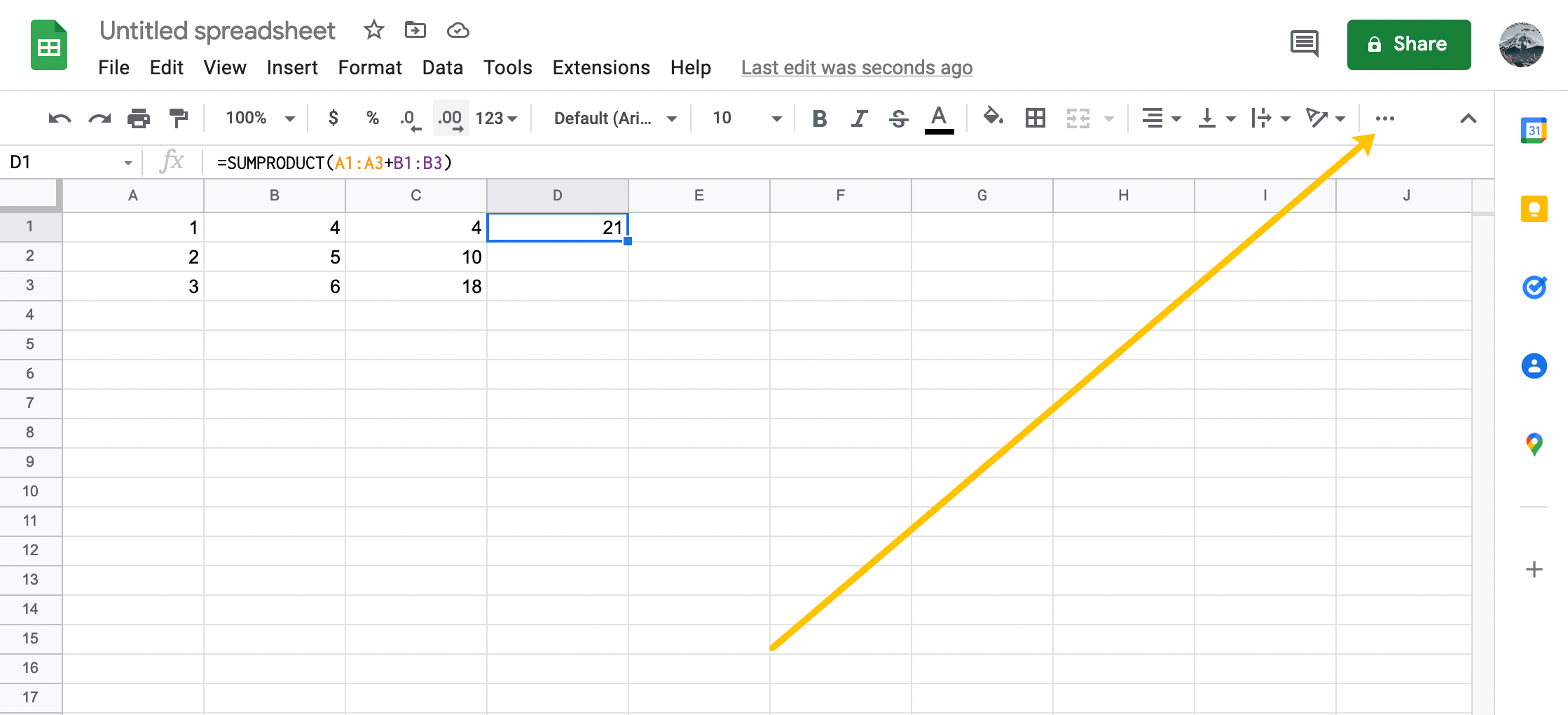2. Click the Chart icon.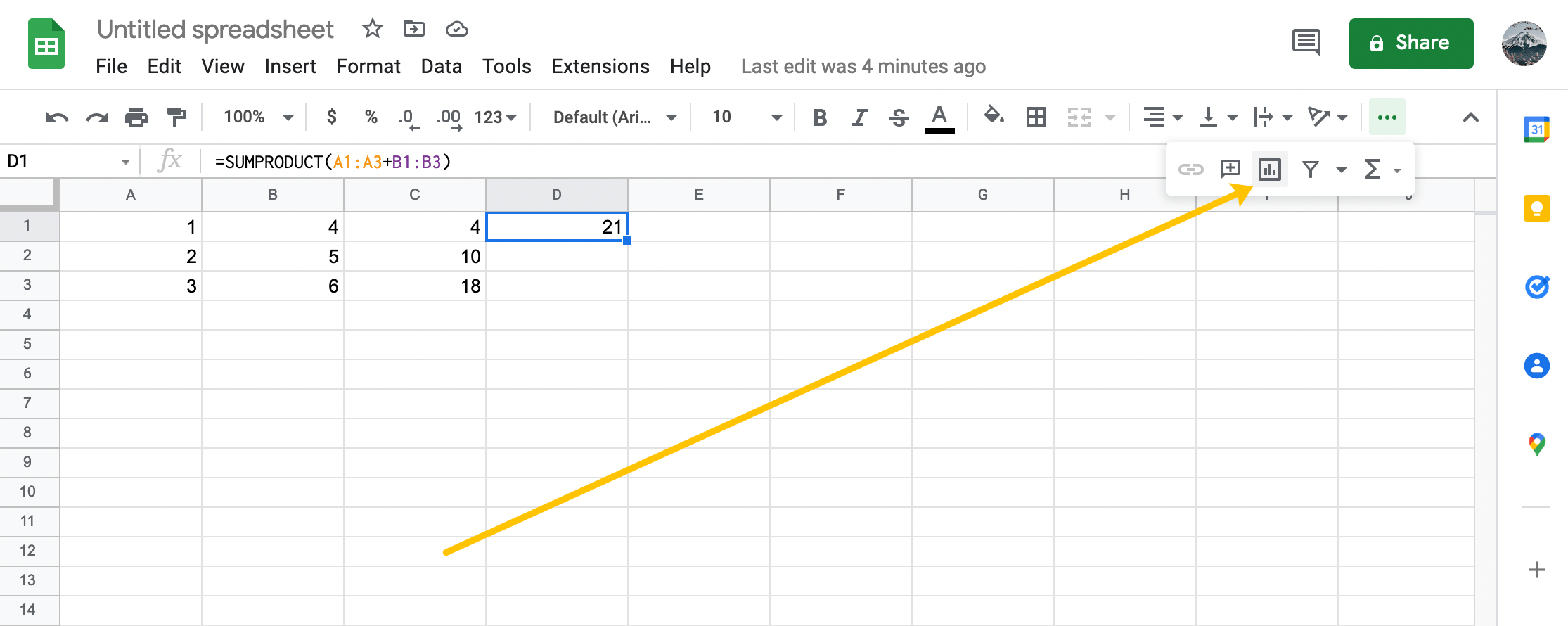It goes down in some places and up in others! How are you supposed to figure the slope of a crazy line like that? The answer is something called a trendline. A trendline is the smoothed-out version of your line that shows the overall trend in the numbers.
3. Double-click on your chart to reveal the sub-menu on the right. Now, select Chart Type.4. Click Scatter Chart located near the bottom.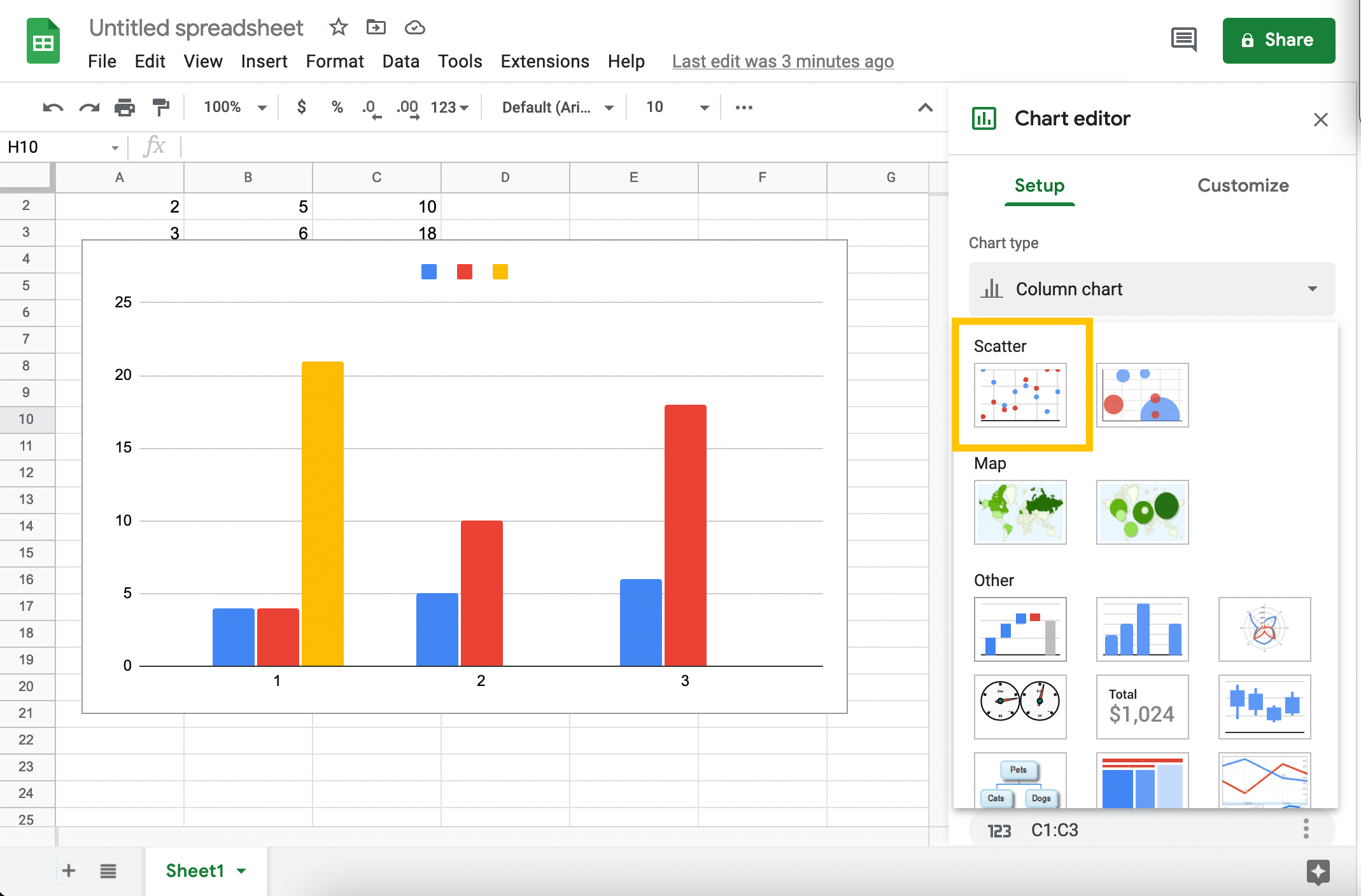5. Click Customize.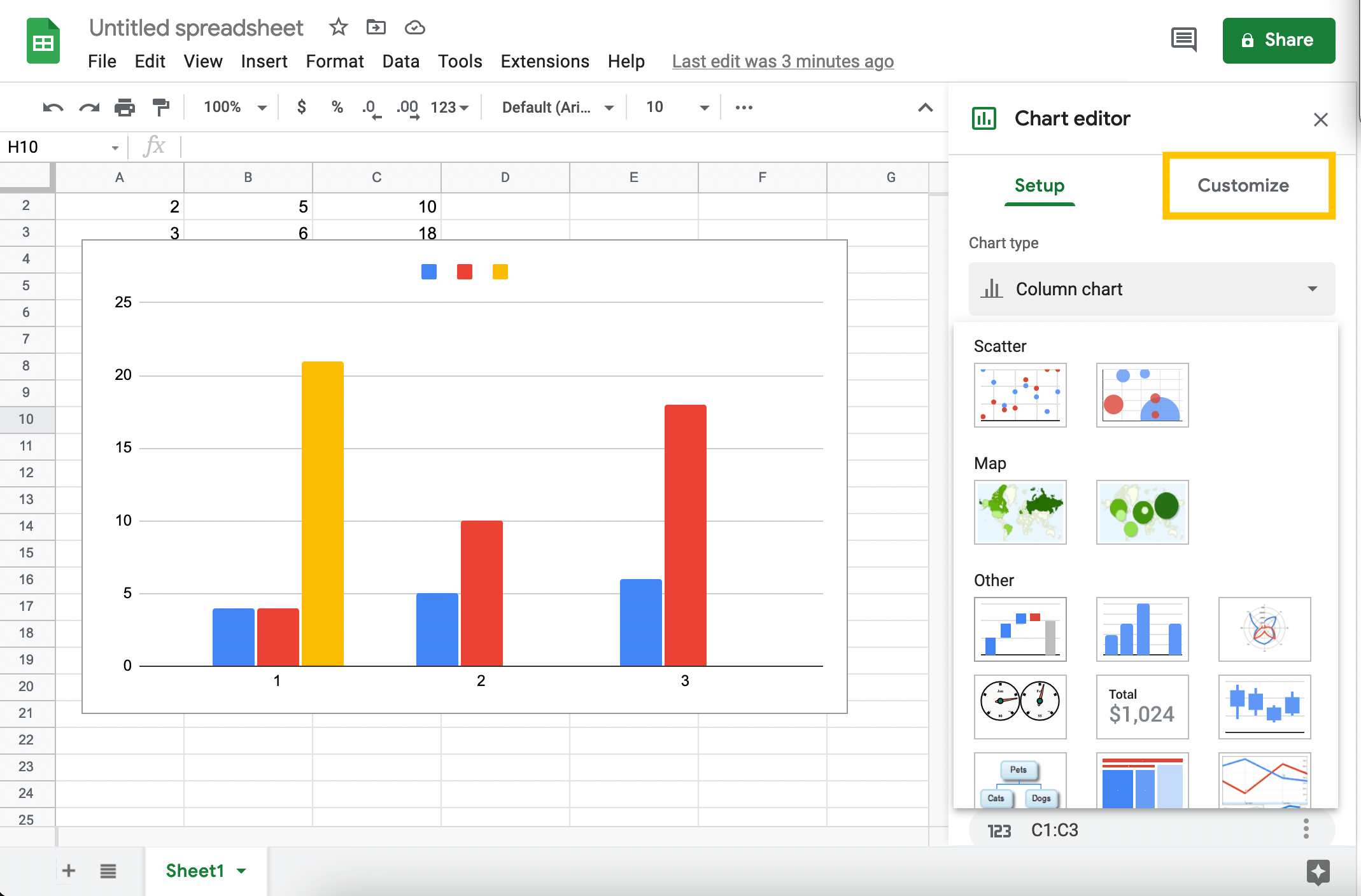6. Click the box next to Trend line.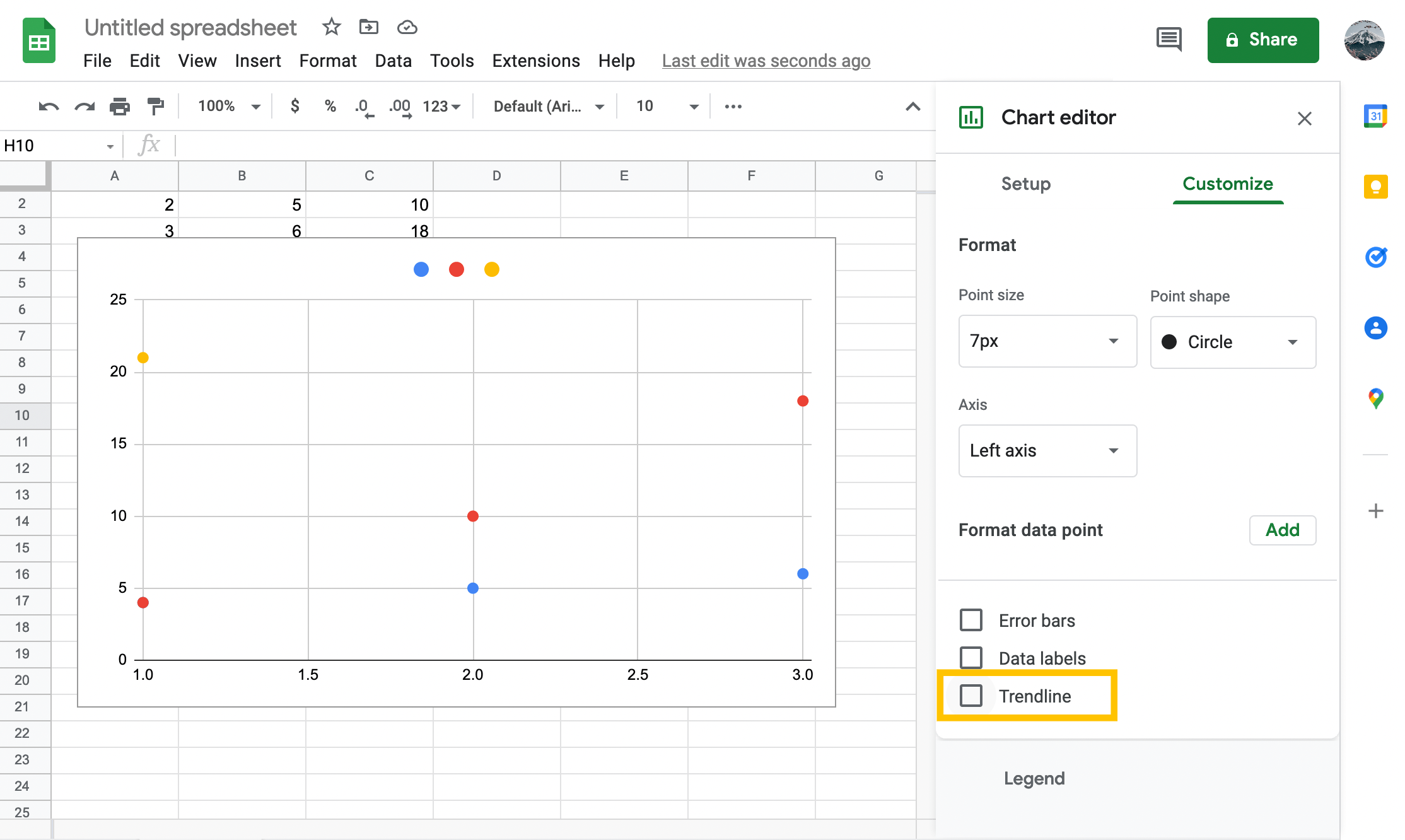Now, your chart should look like this:

The light blue line that follows the string of dots across the chart is the trendline.

So how do you find the slope of that line?

Well, if this were math class, you would have to do some math. Fortunately, this is the 21st century, and math class is well behind us. Instead, we can just tell the computer to do it for us. Thanks, Google.

## How to Find the Slope of a Graph on Google Sheets

We can use Google Sheets to figure the slope within the Chart Editor. Just follow these instructions to find the slope of any line graph in Google Sheets.

1. Select Label > Use Equation. That will add the equation that Google Sheets used to calculate the trendline, and the slope of our line is the part to the left of the *x term.
2. In this case, the slope is +1251. This means that for every one month that passes, the sales revenue increases by a total of \$1,251.

3. Interestingly, you don’t actually have to have a chart to figure out the slope. Google Sheets has a SLOPE function that will calculate the slope of any data table without bothering to draw it as a picture first. Drawing the pictures is very helpful in learning how to do all this, though, which is why we did it that way.

4. Instead of creating the chart, you can just add the SLOPE function to a cell in your spreadsheet. The syntax for Google Sheets’ SLOPE function is SLOPE(data_y, data_x). That function will return the same slope value as in the graph’s equation.

Note that the order of entry is a little bit backward from the way you probably display the information in your table. This is because Sheets wants you to put the independent data (the sales revenue) first and the dependent variable (the month) second.

You should also note that the SLOPE function is not as smart as the chart creator. It needs pure numeric data for the dependent variable, so we’ve changed those cells to be one through 15.

Select any empty cell in the spreadsheet and enter ‘=SLOPE(b2:b16, a2:a16)‘ and hit Return.

And there’s our slope, with a little more precision than the chart provided.

## Final Thoughts

So that’s how you can find slope in Google Sheets. Hopefully, if you were having trouble figuring this out on your own, these instructions were able to help you.

If you prefer to use Excel instead of Sheets, there’s also a TechJunkie guide to finding slope values in Excel.

Do you have interesting applications for finding the slope in Google Sheets? Share them with us below!

Disclaimer: Some pages on this site may include an affiliate link. This does not effect our editorial in any way.

Todays Highlights